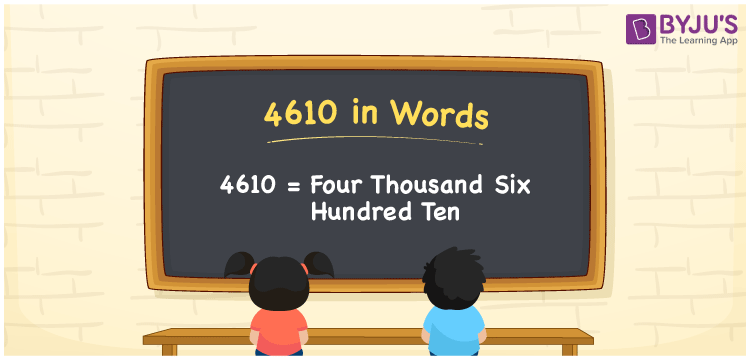# 4610 in Words

In English, the number 4610 is represented as Four Thousand Six Hundred Ten. For instance, If you paid a bus fee of Rs. 4610, then you can say, “I paid a bus fee of Rupees Four Thousand Six Hundred Ten”. 4610 is a cardinal number since it conveys a specific quantity. The number name for 4610 is written using ones, tens, hundreds, thousands places of the number. Hence, a place value chart helps a lot to represent the numerical names. We will learn how to transform the number 4610 into words with a complete explanation in this article.

 4610 in Words Four Thousand Six Hundred Ten Four Thousand Six Hundred Ten in numerical form 4610

## 4610 in English Words

Generally, we write the numbers in words with the help of the English alphabet. Therefore, we can spell and write the number 4610 in English as Four Thousand Six Hundred Ten.## How to Write 4610 in Words?

First, we should determine the place value of each digit in the given number before writing its number name. The following chart shows the place value chart for the number 4610.

 Thousands Hundreds Tens Ones 4 6 1 0

Therefore, we can write the expanded form as:

4 x Thousand + 6 x Hundred + 1 x Ten + 0 x One

= 4 x 1000 + 6 x 100 + 1 x 10 + 0 x 1

= 4000 + 600 + 10 + 0

= 4000 + 600 + 10

= 4610

= Four Thousand Six Hundred Ten

Hence, 4610 in words is written as Four Thousand Six Hundred Ten

Interesting way of writing 4610 in words

4 = Four

46 = Forty-Six

461 = Four Hundred and Sixty-One

4610 = Four Thousand Six Hundred Ten

Thus, the word form of the number 4610 is Four Thousand Six Hundred Ten

4610 is a natural number that is the successor of 4609 and the predecessor of 4611

• 4610 in words – Four Thousand Six Hundred Ten
• Is 4610 an odd number? – No
• Is 4610 an even number? – Yes
• Is 4610 a perfect square number? – No
• Is 4610 a perfect cube number? – No
• Is 4610 a prime number? – No
• Is 4610 a composite number? – Yes

## Frequently Asked Questions on 4610 in Words

Q1

### Write 4610 in words.

4610 in words is Four Thousand Six Hundred Ten.
Q2

### Simplify 4000 + 610, and express in words.

Simplifying 4000 + 610, we get 4610. The number 4610 in words is Four Thousand Six Hundred Ten.
Q3

### Convert Four Thousand Six Hundred Ten in numbers.

Four Thousand Six Hundred Ten in numbers is 4610.